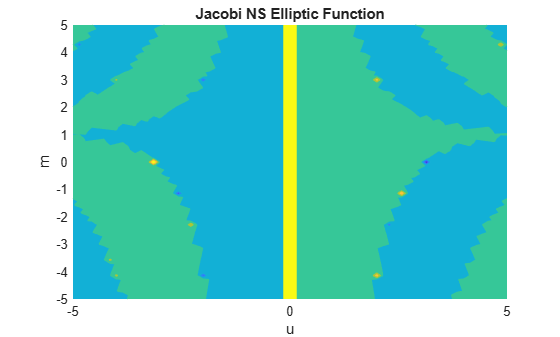jacobiNS

Jacobi NS elliptic function

Description

example

jacobiNS(u,m) returns the Jacobi NS Elliptic Function of u and m. If u or m is an array, then jacobiNS acts element-wise.

Examples

collapse all

jacobiNS(2,1)
ans =
1.0373

Call jacobiNS on array inputs. jacobiNS acts element-wise when u or m is an array.

jacobiNS([2 1 -3],[1 2 3])
ans =
1.0373    1.4879    1.7321

Convert numeric input to symbolic form using sym, and find the Jacobi NS elliptic function. For symbolic input where u = 0 or m = 0 or 1, jacobiNS returns exact symbolic output.

jacobiNS(sym(2),sym(1))
ans =
coth(2)

Show that for other values of u or m, jacobiNS returns an unevaluated function call.

jacobiNS(sym(2),sym(3))
ans =
jacobiNS(2, 3)

For symbolic variables or expressions, jacobiNS returns the unevaluated function call.

syms x y
f = jacobiNS(x,y)
f =
jacobiNS(x, y)

Substitute values for the variables by using subs, and convert values to double by using double.

f = subs(f, [x y], [3 5])
f =
jacobiNS(3, 5)
fVal = double(f)
fVal =
32.1081

Calculate f to higher precision using vpa.

fVal = vpa(f)
fVal =
32.108111189955611054545195854805

Plot the Jacobi NS elliptic function using fcontour. Set u on the x-axis and m on the y-axis by using the symbolic function f with the variable order (u,m). Fill plot contours by setting Fill to on.

syms f(u,m)
f(u,m) = jacobiNS(u,m);
fcontour(f,'Fill','on')
title('Jacobi NS Elliptic Function')
xlabel('u')
ylabel('m')Input Arguments

collapse all

Input, specified as a number, vector, matrix, or multidimensional array, or a symbolic number, variable, vector, matrix, multidimensional array, function, or expression.

Input, specified as a number, vector, matrix, or multidimensional array, or a symbolic number, variable, vector, matrix, multidimensional array, function, or expression.

collapse all

Jacobi NS Elliptic Function

The Jacobi NS elliptic function is

ns(u,m) = 1/ds(u,m)

where ds is the respective Jacobi elliptic function.

The Jacobi elliptic functions are meromorphic and doubly periodic in their first argument with periods 4K(m) and 4iK'(m), where K is the complete elliptic integral of the first kind, implemented as ellipticK.